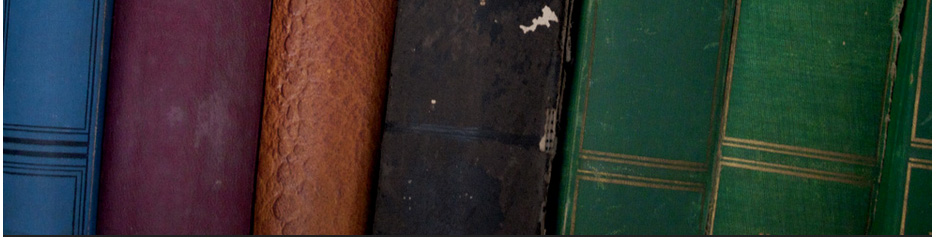## Secondary educationks3 Maths video collections

Geometry

ks30001 Angles in a Triangle

ks30002 Finding missing angles in triangles and parallel lines 10A2

ks30003  BASIC Maths - How to Work Out Angles

ks30004 Missing Angles in Isosceles

ks30005 Triangle Congruence - SSS, SAS, ASA and AAS

ks30006 Triangle Congruence Theorems, Two Column Proofs, SSS, SAS, ASA, AAS, Geometry Practice Problems

ks30007 Coordinate Geometry - Equation of a line (1) : ExamSolutions

ks30008  Coordinate Geometry Basic Concepts

ks30009 Coordinate Geometry

ks30010 Solving Simultaneous Equations

ks30011 Coordinate Geometry Definitions & Formulas

ks30012 Circle Theorems made easy

ks30013  Circle Theorems

ks30014 Circle theorems

ks30015 Circle theorems

Pythagoras & Trigonometry

ks30001 Pythagoras & Trigonometry Revision Mathscast - YouTube

ks30002 Pythagoras | Trigonometry

ks30003   Pythagoras' Theorem or Trigonometry - which one?

ks30005 Pythagoras Theorem

Ermias Home

HAREP The Horn of Africa Research and Knowledge Exchange Platform © 2010-2012

 Contact: rkidane@talk21.com Please click Here to send your feedback